×

### Graphing Calculator Not Up-to-date

uCalc Graphing Calculator was released years ago, and relied on features no longer supported in Windows 7 and beyond. At startup, you will get an error message. Once you get past the error message the calculator will work. The part that won't work is the animated cartoon character used in the tutorial. You may want to consider using uCalc Console Calculator instead for now.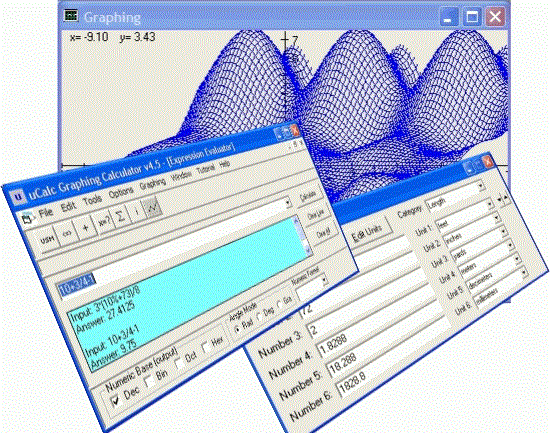If you are a student who wants to plot those equations the teacher gave for math assignment, or an engineer looking for a great scientific calculator, or a home user who wants to convert between units of measure (such as teaspoons to tablespoons for that special recipe), or you want to compute the number of mortgage payments that are left by simply filling in the blanks, then this multi-purpose calculator is for you.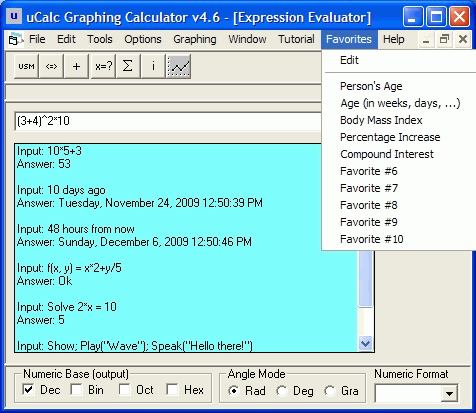Here is the list of main features for the uCalc Graphing Calculator:

• Interactive Animated Tutorial -- Sit back and watch (and interact) as an animated character walks you through many of the calculator's features. You may likewise create your own tutorials to demonstrate math lessons by programming the animated character. (Unfortunately the animation agent was removed from Windows starting with Windows 7)
• Expression Evaluator -- It comes with many built-in functions, operators, numeric bases, numeric formats, and modes.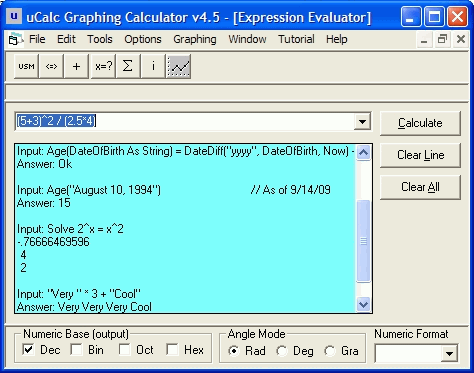• Unit Converter -- You can convert between units of measure in a flexible manner. You'll find categories such as Length, Capacity, Weight, Temperature, Time, and more. You can even add your own categories and units.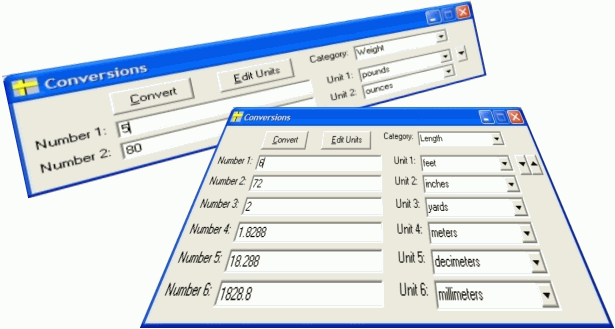• User Solution Modules -- You can interactively define and solve problems by simply filling in the blanks, without directly having to manipulate math formulas. To get you started, uCalc comes with useful financial modules that will let you calculate things like add-on interest, compound interest, ordinary annuity sum, present value (mortgage), and more. You might find yourself eventually creating your own modules to solve all kinds of other problems with this unique feature.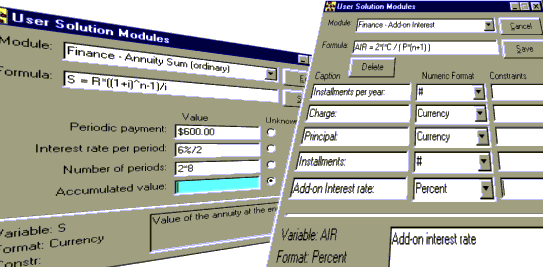• Graphing -- You can plot Cartesian, polar, parametric, 3D equations, or coordinates from data files. You can print your graphs, or save them for later use.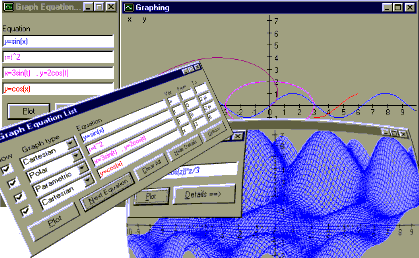• Equation Solver -- You can solve for the unknown value in an equation. If your equation has several solutions, this calculator can display them all. If there are several solutions, but you are interested in only the negative ones for instance, you can enter the solution range you want uCalc to work in.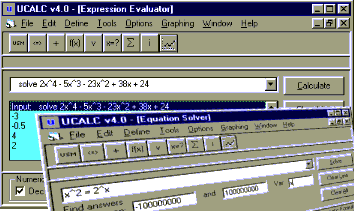• User Functions & Variables -- You can define variables with meaningful names, and functions with multiple parameters for use anywhere in the program.
• Summation Tables -- You can use this general mathematical tool for practical things such as calculating annuity, compound growth, etc...
• Integrator -- You can do numerical integrations as well.